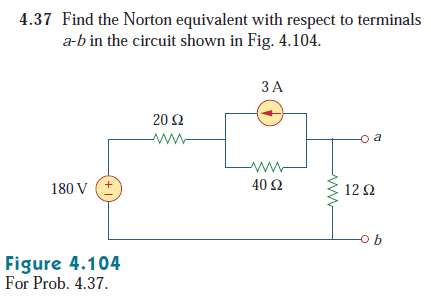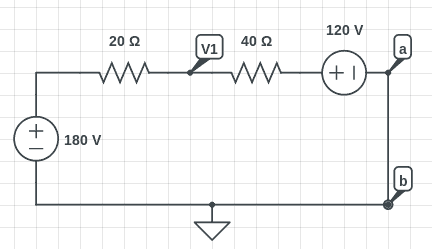# [Circuits] Calculating the Norton Equivalent

• Engineering

## Homework Statement## The Attempt at a Solution

I am trying to compute ##I_N##.

I connected nodes a and b. The circuit after some simplification:$$\frac { 180-{ V }_{ 1 } }{ 20 } =\frac { 120-V_{ 1 } }{ 40 } \\ { V }_{ 1 }=240\quad V\\ \\ R=\frac { V }{ I } \\ 40=\frac { 120 }{ I_{ N } } \\ I_{ N }=3\quad A$$

Last edited:

phinds
Gold Member
Why would you replace the 3amp current source with a 120 volt voltage source (I KNOW why you did it, but it's wrong and I want you to think about it)

Why would you replace the 3amp current source with a 120 volt voltage source (I KNOW why you did it, but it's wrong and I want you to think about it)

I did it to simplify the circuit and make the nodal analysis easier.

gneill
Mentor
Actually, changing the 3A / 40Ω subcircuit to its Thevenin equivalent works here. You're left with a simple series circuit, so no need for nodal analysis. Just calculate the current from the given resistances and voltages.

Actually, changing the 3A / 40Ω subcircuit to its Thevenin equivalent works here. You're left with a simple series circuit, so no need for nodal analysis. Just calculate the current from the given resistances and voltages.

Is there something wrong with my first attempt?

gneill
Mentor
Is there something wrong with my first attempt?

Yes. Where did the 240V come from ?

Yes. Where did the 240V come from ?

I typed it wrong. I have fixed it now. Sorry about that.

gneill
Mentor
I typed it wrong. I have fixed it now. Sorry about that.

Okay, now you need to correct the signs in your node equation. The left hand side yields a current flowing into the node from the 180V supply. On the right hand side you should therefore have a current flowing from the node to the 120V supply. You've got the right hand side yielding a current flowing from the 120V supply to the V1 node instead.

I find it easier, and less prone to error, to write all node equations from the perspective of the node itself and assuming that all currents are flowing out of the node (my choice of direction; you could choose the opposite if you wish). Sum all these currents on the same side of the equals and equate to zero:

##\frac{V1 - 180}{20} + \frac{V1 - 120}{40} = 0##

•1 person
Okay, now you need to correct the signs in your node equation. The left hand side yields a current flowing into the node from the 180V supply. On the right hand side you should therefore have a current flowing from the node to the 120V supply. You've got the right hand side yielding a current flowing from the 120V supply to the V1 node instead.

I find it easier, and less prone to error, to write all node equations from the perspective of the node itself and assuming that all currents are flowing out of the node (my choice of direction; you could choose the opposite if you wish). Sum all these currents on the same side of the equals and equate to zero:

##\frac{V1 - 180}{20} + \frac{V1 - 120}{40} = 0##

Ah, that makes sense. Yeah, I think I'm going to adopt the same convention to avoid these types of mistakes again.